# Selina Concise Mathematics Class 9 ICSE Solutions Complementary Angles

## Selina Concise Mathematics Class 9 ICSE Solutions Complementary Angles

APlusTopper.com provides step by step solutions for Selina Concise Mathematics Class 9 ICSE Solutions Chapter 25 Complementary Angles. You can download the Selina Concise Mathematics ICSE Solutions for Class 9 with Free PDF download option. Selina Publishers Concise Mathematics for Class 9 ICSE Solutions all questions are solved and explained by expert mathematic teachers as per ICSE board guidelines.

Selina ICSE Solutions for Class 9 Maths Chapter 25 Complementary Angles

Exercise 25(A)

Solution 1(i):Solution 1(ii):Solution 1(iii):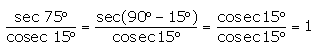Solution 1(iv):Solution 1(v):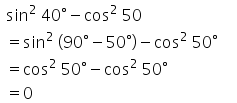Solution 1(vi):Solution 1(vii):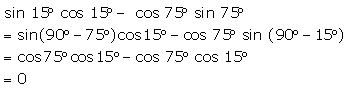Solution 1(viii):Solution 2(i):Solution 2(ii):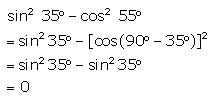Solution 2(iii):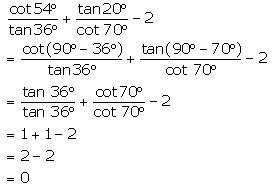Solution 2(iv):Solution 2(v):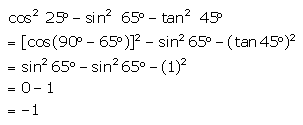Solution 2(vi):Solution 3(i):Solution 3(ii):Solution 4:Solution 5: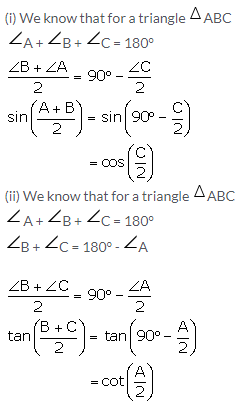Solution 6: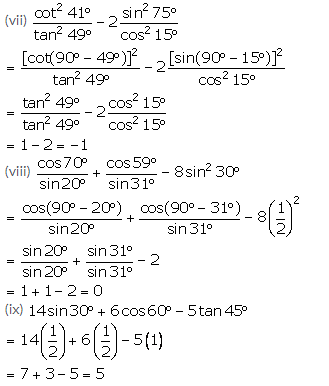Solution 7:Solution 8(i):Solution 8(ii):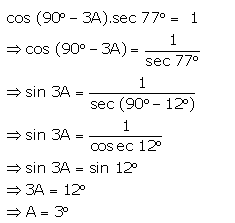More Resources for Selina Concise Class 9 ICSE Solutions